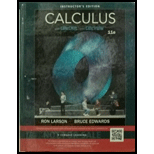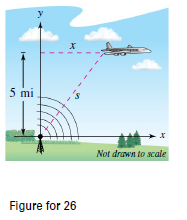Chapter 2.6, Problem 26E### Calculus (MindTap Course List)

11th Edition
Ron Larson + 1 other
ISBN: 9781337275347

#### Solutions

Chapter
Section### Calculus (MindTap Course List)

11th Edition
Ron Larson + 1 other
ISBN: 9781337275347
Textbook Problem

# Air Traffic Control An airplane is flying at an altitude of 5 miles and passes directly over a radar antenna (see figure). When the plane is 10 miles away ( s = 10 ) , the radar detects that the distance 5 is changing at a rate of 240 miles per hour. What is the speed of the plane?To determine

To calculate: The speed of the plane when the plane is 10 miles away from the radar and its distance from the radar is changing at a rate of 240 miles per hour.

Explanation

Given:

An airplane is flying at an altitude of5 miles and passes directly over a radar antenna as shown in the figure. When the plane is 10 miles away, the radar detectsthat the distance s is changing at a rate of 240 miles per hour.

Formula used:

The Pythagoras theorem for a right-angled triangle with hypotenuse h and other two sides, a,b is given by a2+b2=h2.

If, y=f(u) is a differentiable function of u and u=g(x) is a differentiable function of x, then y=f(g(x)) is a differentiable function of x and,

dydx=dydududx

The derivative of the function, xn is:

ddx(xn)=nxn1

Calculation:

The rate at which the distance s is changing is:

dsdt=240

The value of s can be calculated by applying Pythagoras theorem in the triangle given below:

Applying the Pythagorean Theorem in the above triangle, we get

s2=x2+52=x2+25

Now, we will differentiate the above equation with respect to t.

ddt(s2)=ddt(x2+52)=ddt(x2)+ddt(25)

The derivative of a constant is zero

### Still sussing out bartleby?

Check out a sample textbook solution.

See a sample solution

#### The Answers to Your Study Problems

Solve them all with bartleby. Boost your grades with guidance from subject experts covering thousands of textbooks. All for just \$9.99/month

Get As ASAP

## Additional Math Solutions

#### Find more solutions based on key concepts